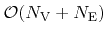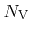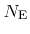Next: 4.8.2 Graph Setup Algorithm Up: 4.8 Void Detection Previous: 4.8 Void Detection

## 4.8.1 Connected Components

The LS function which represents the surface, partitions the simulation domain into connected components. In the following section a fast algorithm is presented which uses inherent properties of the H-RLE data structure to determine the corresponding component each grid point belongs to. The determination of connected components gives information about existing voids. If there are no voids, there are only two components which correspond to the bulk material and the region above the surface as part of the process chamber. The obtained connectivity information can also be used to ensure that the geometry of voids does not change after they have been formed.

Two neighboring grid points are defined to be connected, if and only if they have the same LS sign. If they do not have the same sign, they are separated by the zero LS which is the surface. The connectivity relations between neighboring grid points can be described by a graph, where each grid point corresponds to a vertex. The connectivity of two neighboring grid points is represented by an edge between the corresponding vertices. According to elementary graph theory the connected components of a graph can be determined with a complexity of, whereanddenote the number of vertices and edges, respectively . Obviously, setting up a full graph with vertices for each point on the regular grid is not reasonable, since the memory requirements and the computation of the connected components scale with the domain size and not linearly with the surface size.Next: 4.8.2 Graph Setup Algorithm Up: 4.8 Void Detection Previous: 4.8 Void Detection

Otmar Ertl: Numerical Methods for Topography Simulation